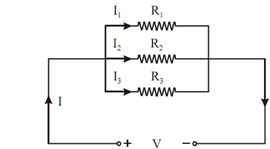## Voltage Division Rule Assignment Help

Assignment Help: >> Voltage and Current Division in Resistive Circuits - Voltage Division Rule

Voltage Division Rule:

Since in series circuit, shown in Figure, current is illustrated by equation

I =  V /Req

then

V1 = IR1 = V.  (R1/Req)

V2 = IR2 = V.  (R2 /Req)

V3 = IR3 = V.  (R3 /Req)

Figure shows the resistance connected is parallel.Figure: Parallel Resistors

In parallel circuits, current is divided whereas the voltage remains same across all parallel branches.

1 /Req =  (1 / R1 )+  (1 / R2) +  (1/ R3)

= (R2 R3  + R1 R3  + R1 R2 )/ R1 R2 R3

∴          Req = R1 R2 R3 / (R1 R2 + R2 R3 + R3 R1)

It is better to represent the circuit in conductance form.

i.e. Geq  = G1 + G2 + G3#### Assured A++ Grade

Get guaranteed satisfaction & time on delivery in every assignment order you paid with us! We ensure premium quality solution document along with free turntin report!

All rights reserved! Copyrights ©2019-2020 ExpertsMind IT Educational Pvt Ltd